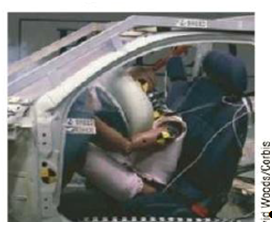Chapter 6, Problem 12CQ

Chapter
Section
Textbook Problem

An air bag inflates when a collision occurs, protecting a passenger (the dummy in Figure CQ6.12) from serious injury. Why does the air bag soften the blow? Discuss the physics involved in this dramatic photograph.To determine
The reasons that air bag soften the blow.

Explanation

The air bag protects the passenger can be explained by using the concept of momentum.

Linear Momentum: The linear momentum p of an object of mass m moving with velocity v is the product of its mass and velocity.

p=mv

• p is the momentum of the object
• m is the mass of the object
• v is the velocity of the object

Impulse: If a constant force F acts on an object, the impulse I delivered to the object over a time interval Δt is given by

I=FΔt

• I is the impulse on an object
• F is the net force acts on an object
• Δt is the time duration through which force acts on an object

Change in momentum: Impulse of the force action on an object equals the change in momentum of that object. Fav is the average force

Still sussing out bartleby?

Check out a sample textbook solution.

See a sample solution

The Solution to Your Study Problems

Bartleby provides explanations to thousands of textbook problems written by our experts, many with advanced degrees!

Get Started

B vitamins often act as: a. antioxidants. b. blood clotting factors. c. coenzymes. d. none of the above.

Nutrition: Concepts and Controversies - Standalone book (MindTap Course List)

How do eating disorders affect health?

Understanding Nutrition (MindTap Course List)

Draw the general block diagram for a biological wax.

General, Organic, and Biological Chemistry